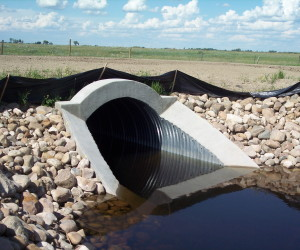# How to Calculate Hydraulic DiameterThe hydraulic diameter is a term used in fluid mechanics and thermodynamics. It is a measure of the efficiency of a section to pass flow by relating the cross-sectional area of flow to the wetted perimeter of the cross-section.

The hydraulic diameter of a round pipe is simply its diameter. The equations for hydraulic diameter for other cross-sections are given in the table below.

Flowing Full
Circle$D$
Annulus$D_{outside} - D_{inside}$
Square (L = side)$L$
Rectangle (L1 and L2 = sides)$\frac{2L_1L_2}{L_1 + L_2}$
Flowing partially full
Half-filled circle (d = diameter)$d$
Rectangle (h = height, w = width)$\frac{4hw}{w + 2h}$
Wide, shallow stream (d = depth)$4d$
Triangle (d = depth, t = top, s = side length)$\frac{d\cdot t}{s}$
Trapezoid (d = depth, t = top, b = bottom, s = side length)$\frac{2d(t + b)}{b + 2s}$

The hydraulic diameter should not to be confused with the hydraulic radius term in the Manning equation, which is not half of the hydraulic diameter, but rather one quarter.

This site uses Akismet to reduce spam. Learn how your comment data is processed.